# R S Aggarwal Solutions for Class 10 Maths Chapter 16 Area of Circle, Sector and Segment Exercise 16A

R S Aggarwal Solutions for Class 10 Maths Chapter 16 Area of Circle, Sector and Segment exercise 16A is provided here. Exercise 16A helps students in solving problems based on area of circle, sector and segment. All answers are prepared by subject experts using diagrams for better understanding. Download free R S Aggarwal Solutions of Class 10 Chapter 16 pdf for Maths and sharpen your skills.

## Download PDF of R S Aggarwal Solutions for Class 10 Maths Chapter 16 Area of Circle, Sector and Segment Exercise 16A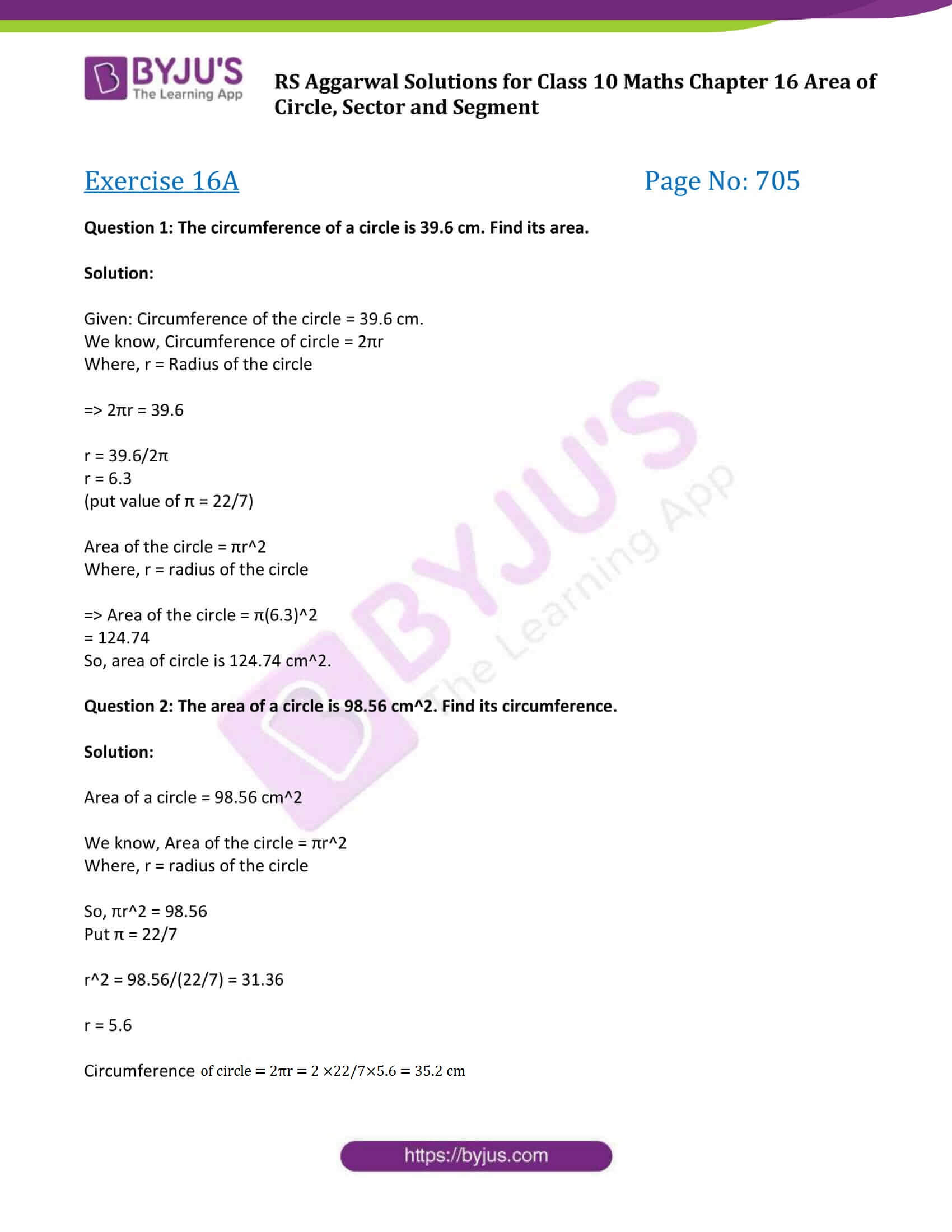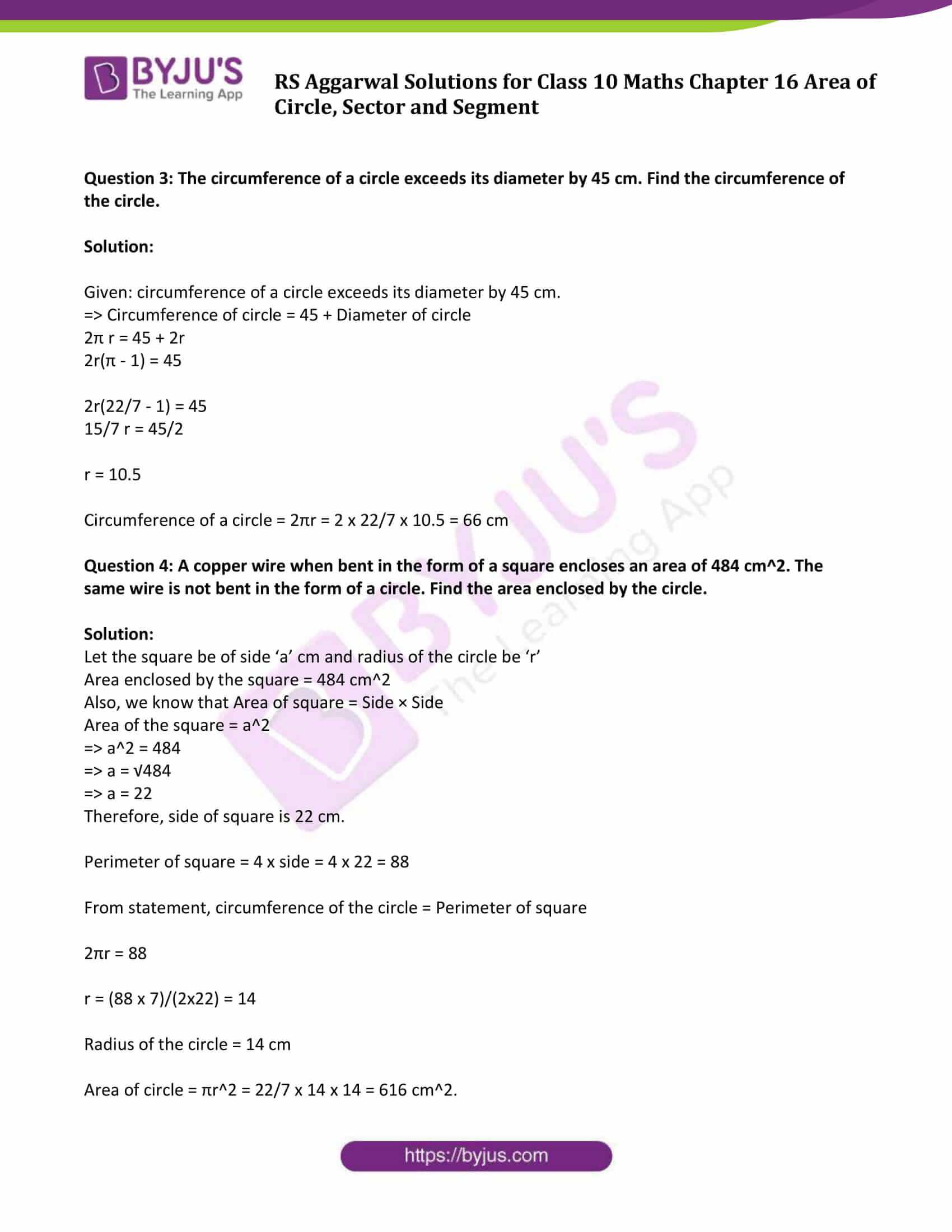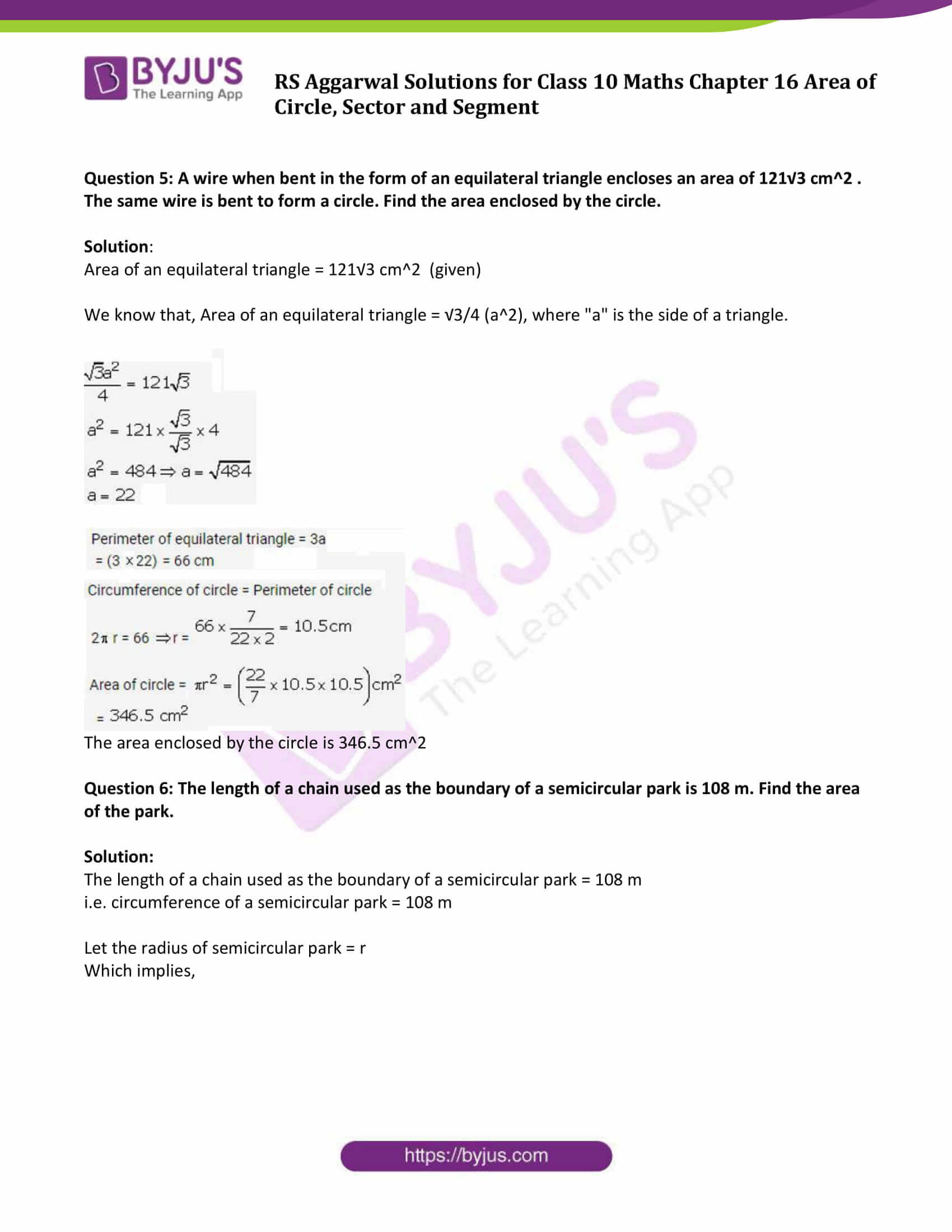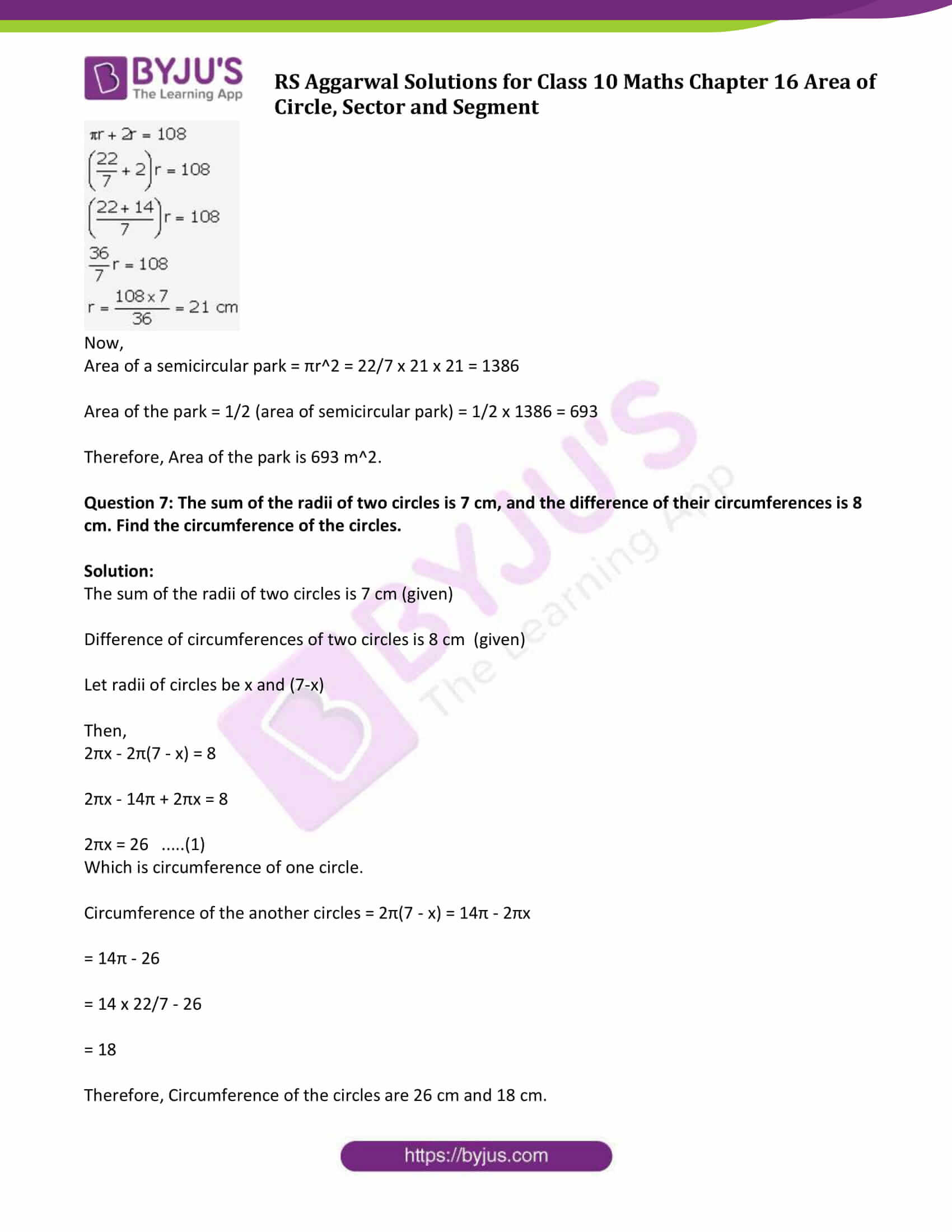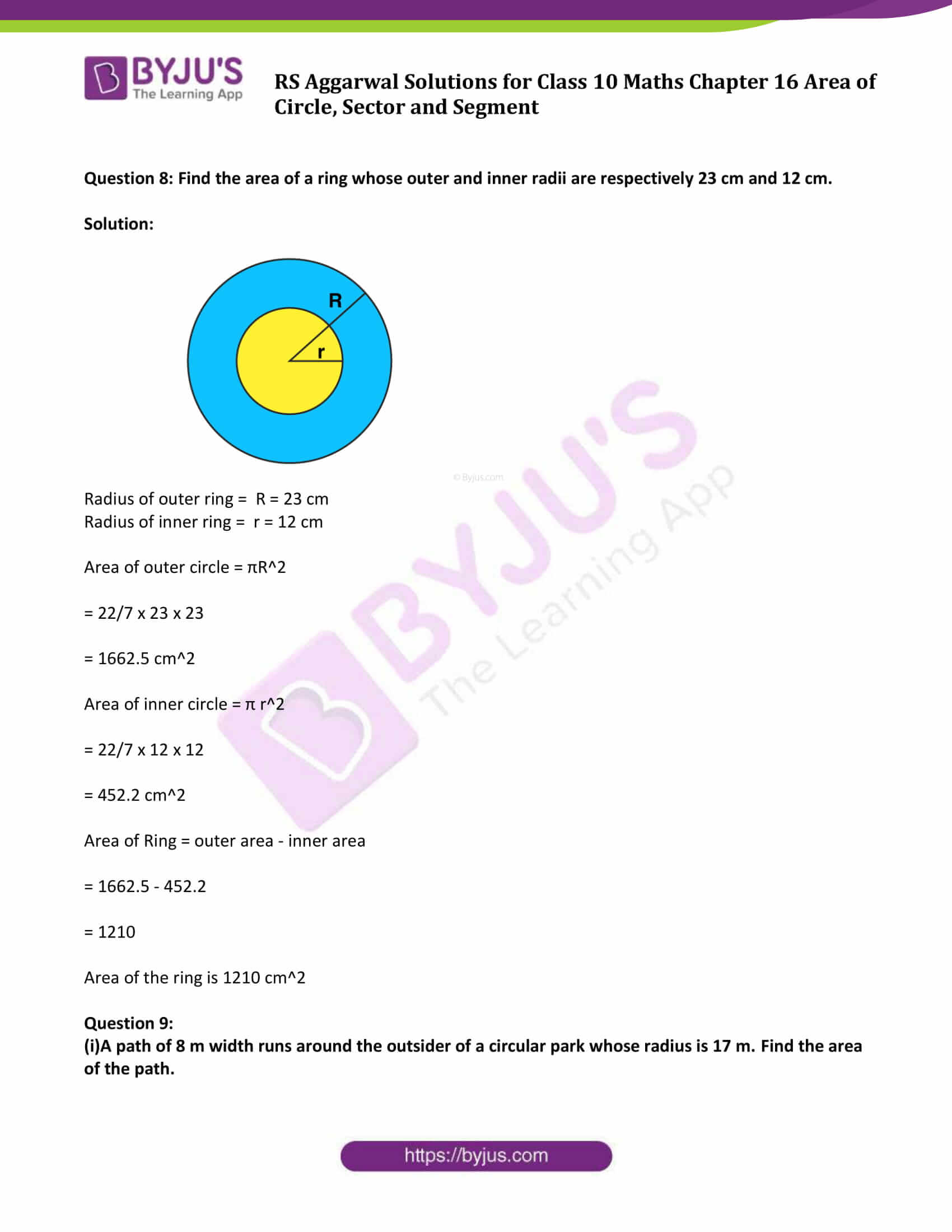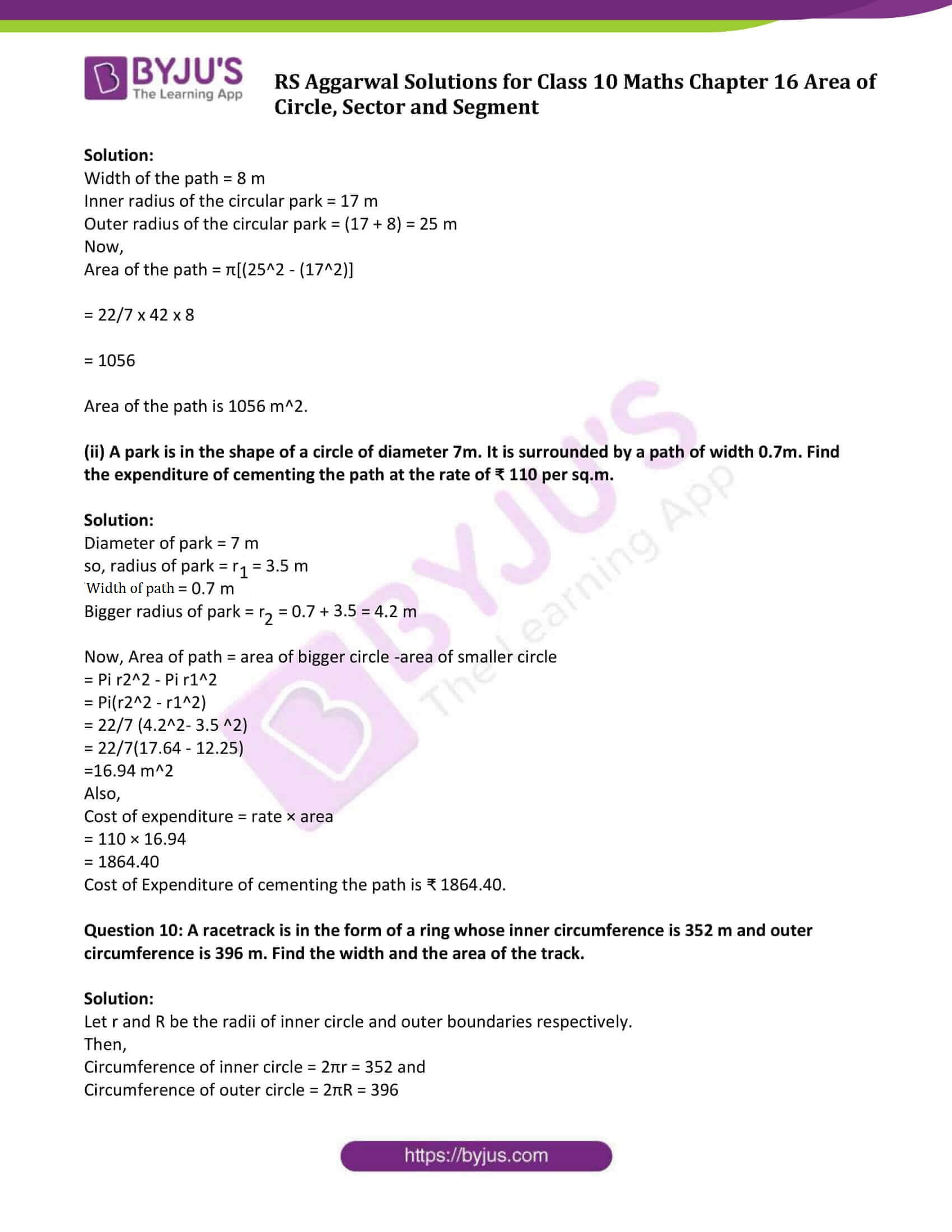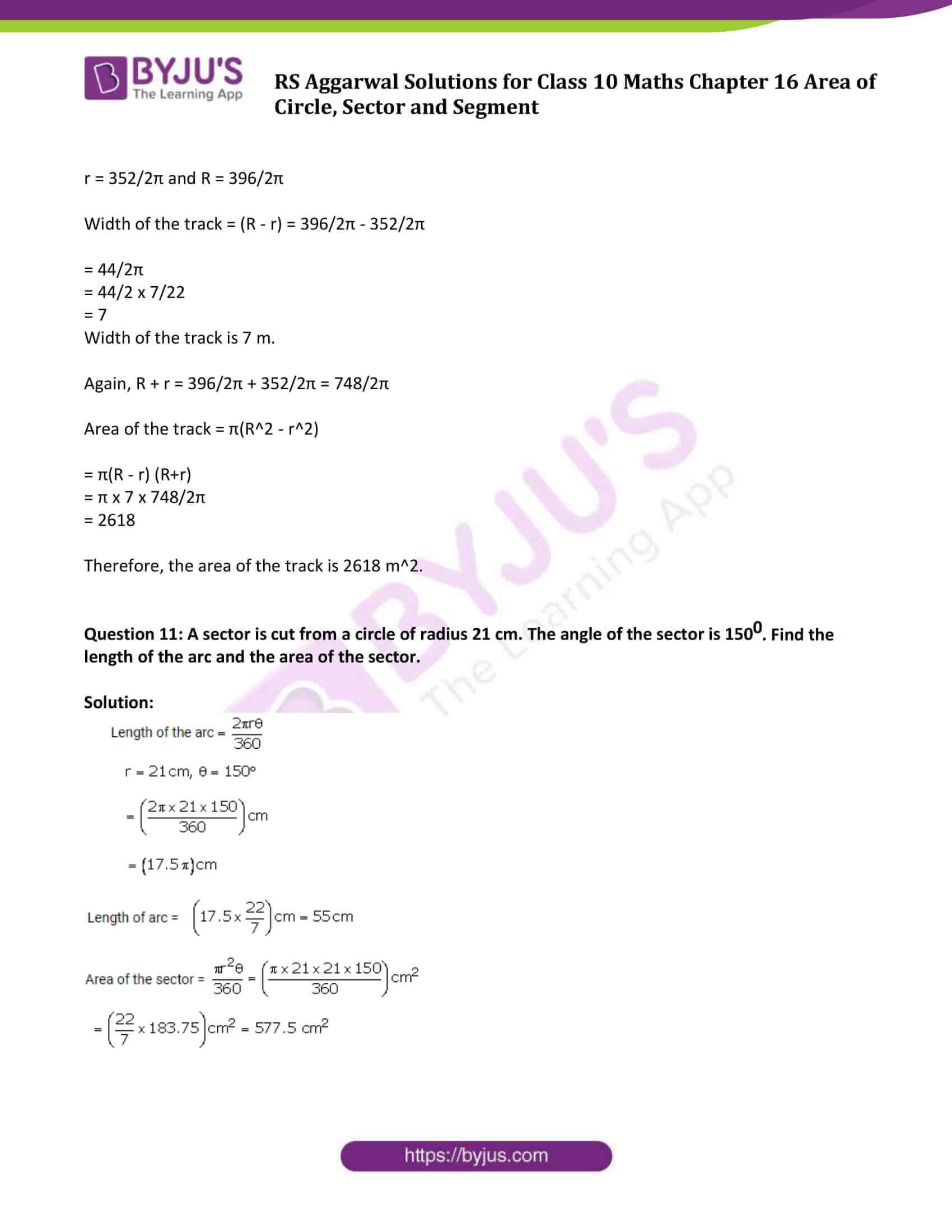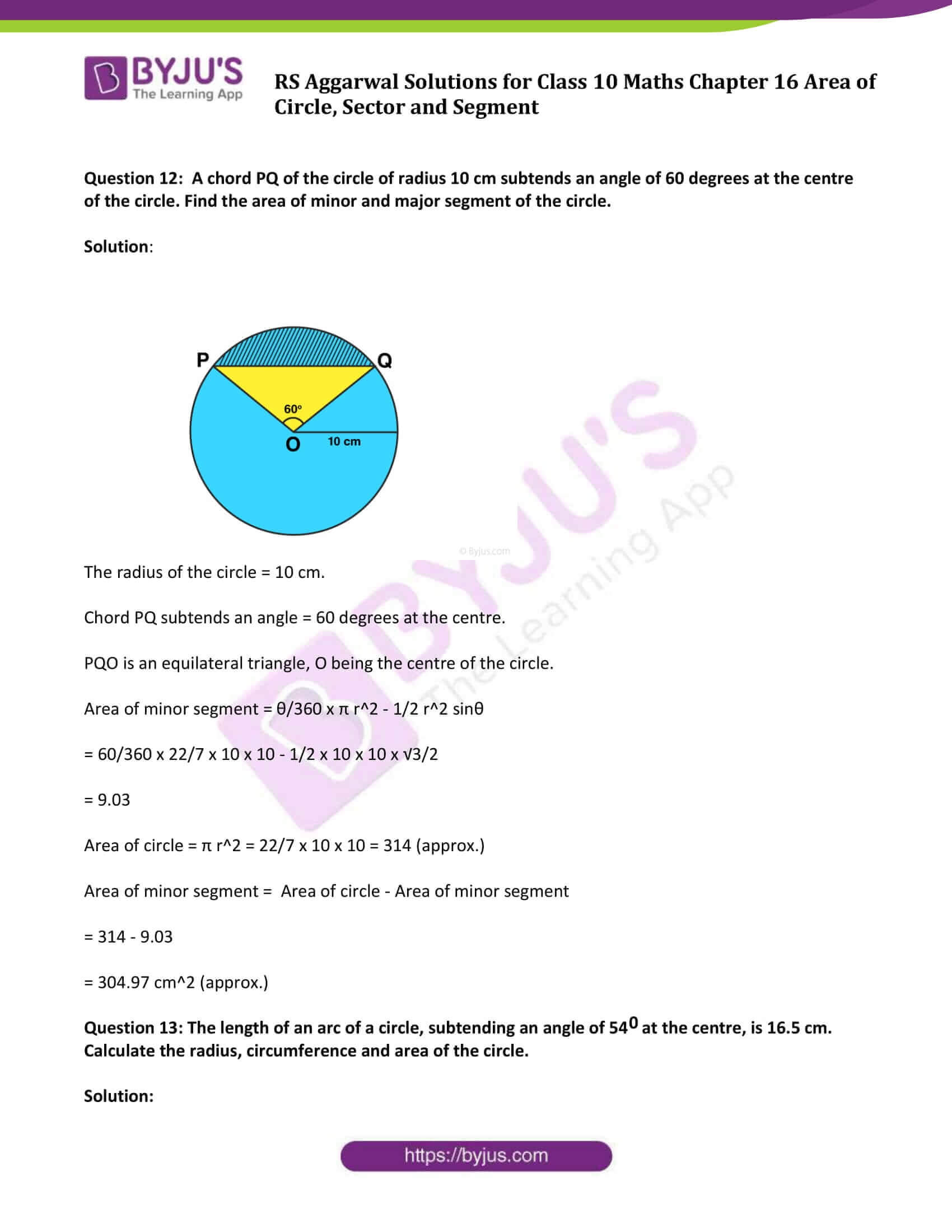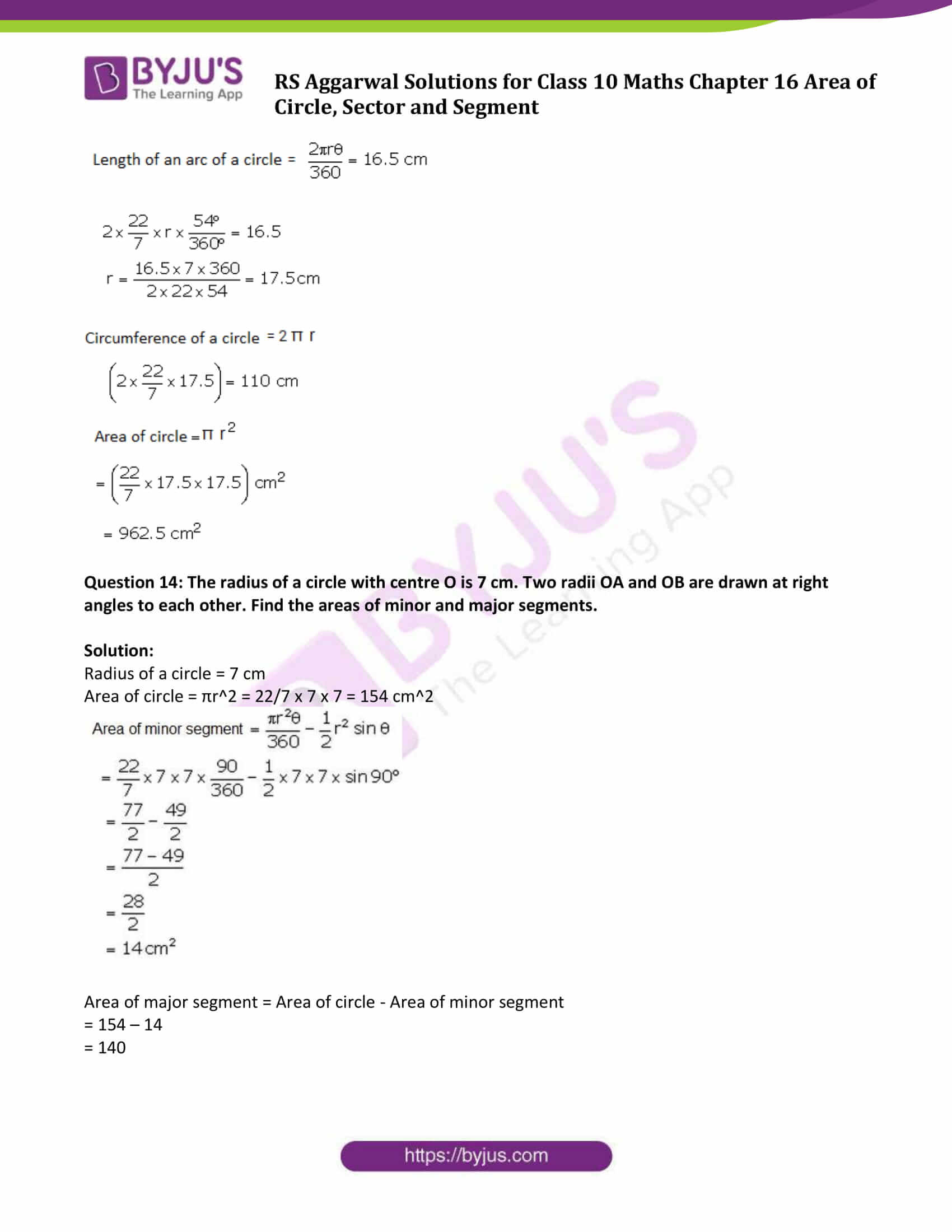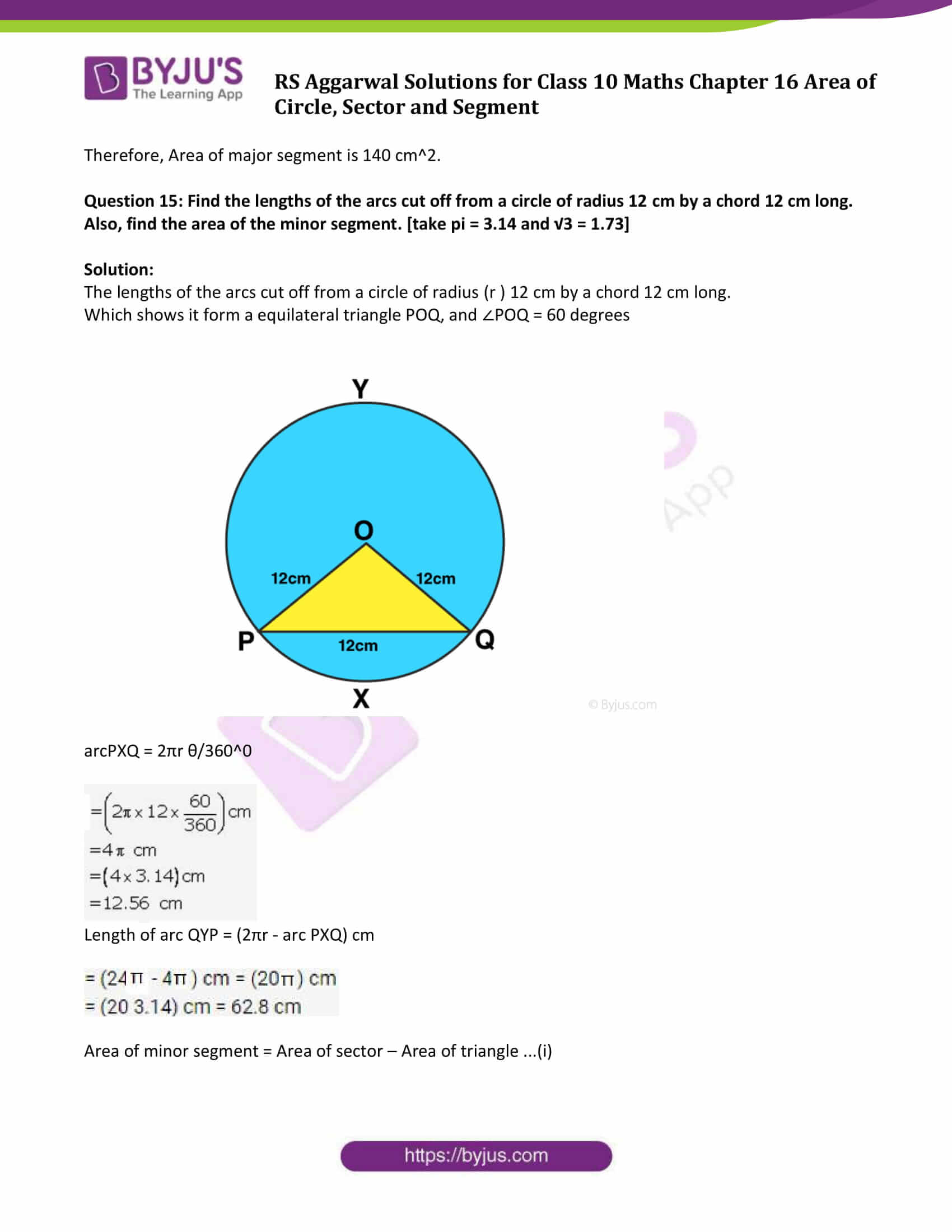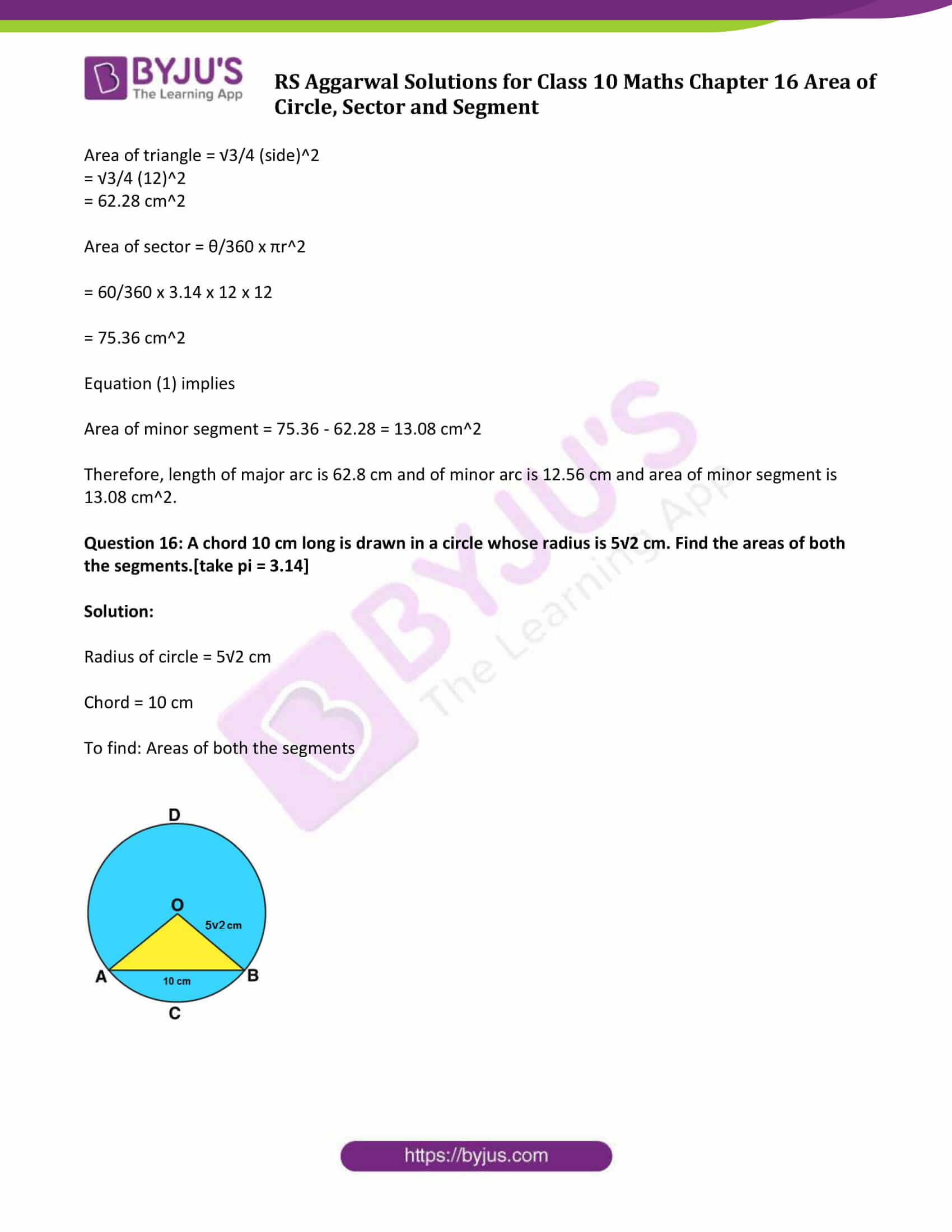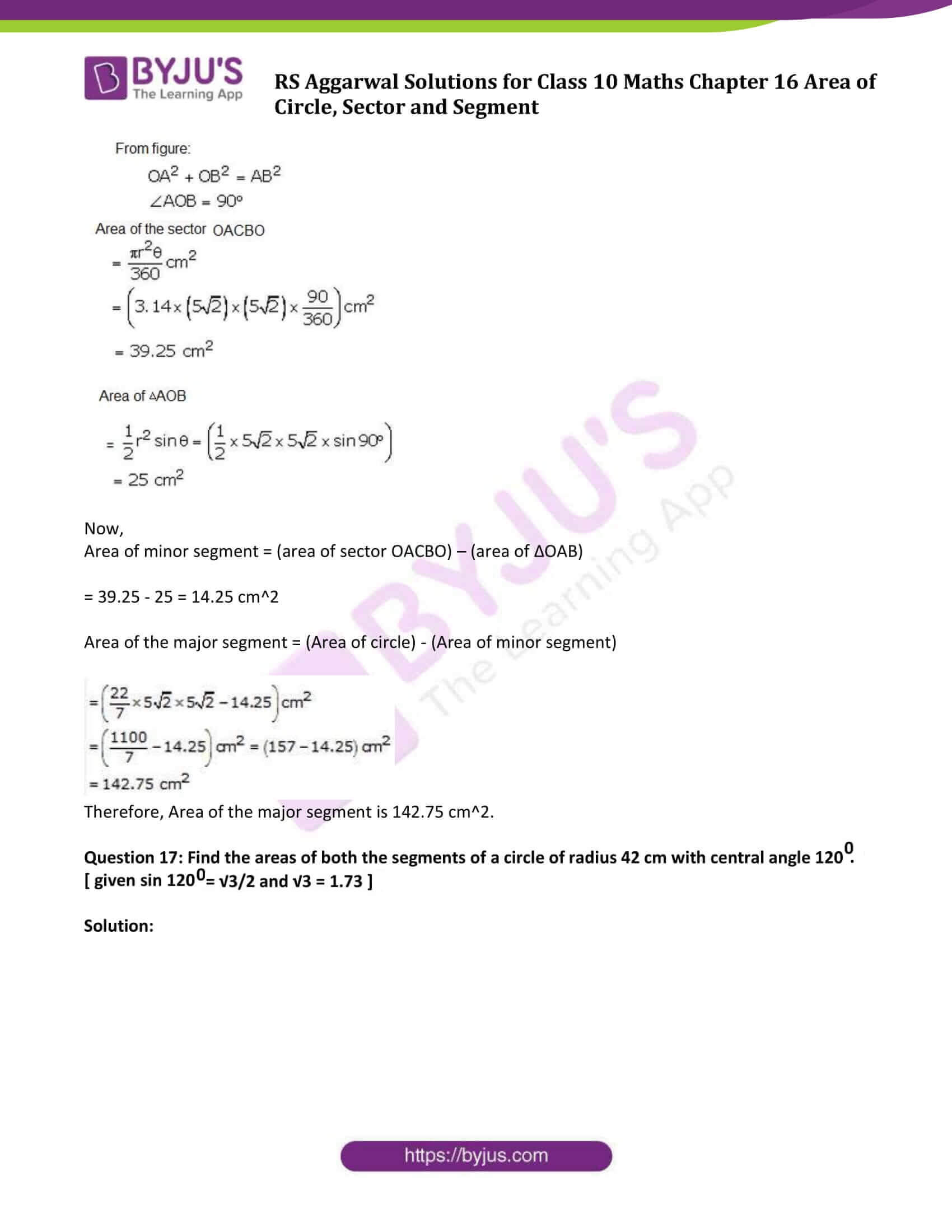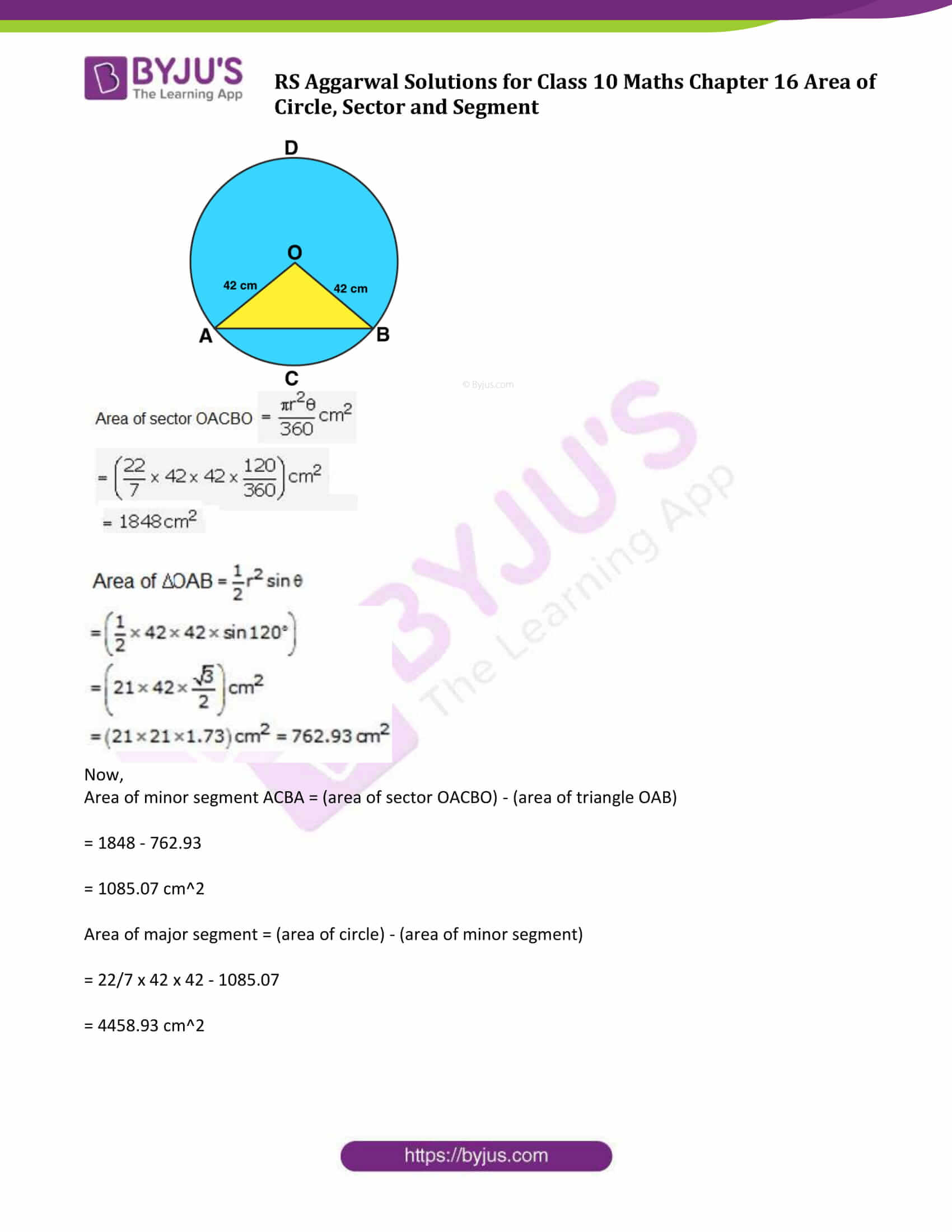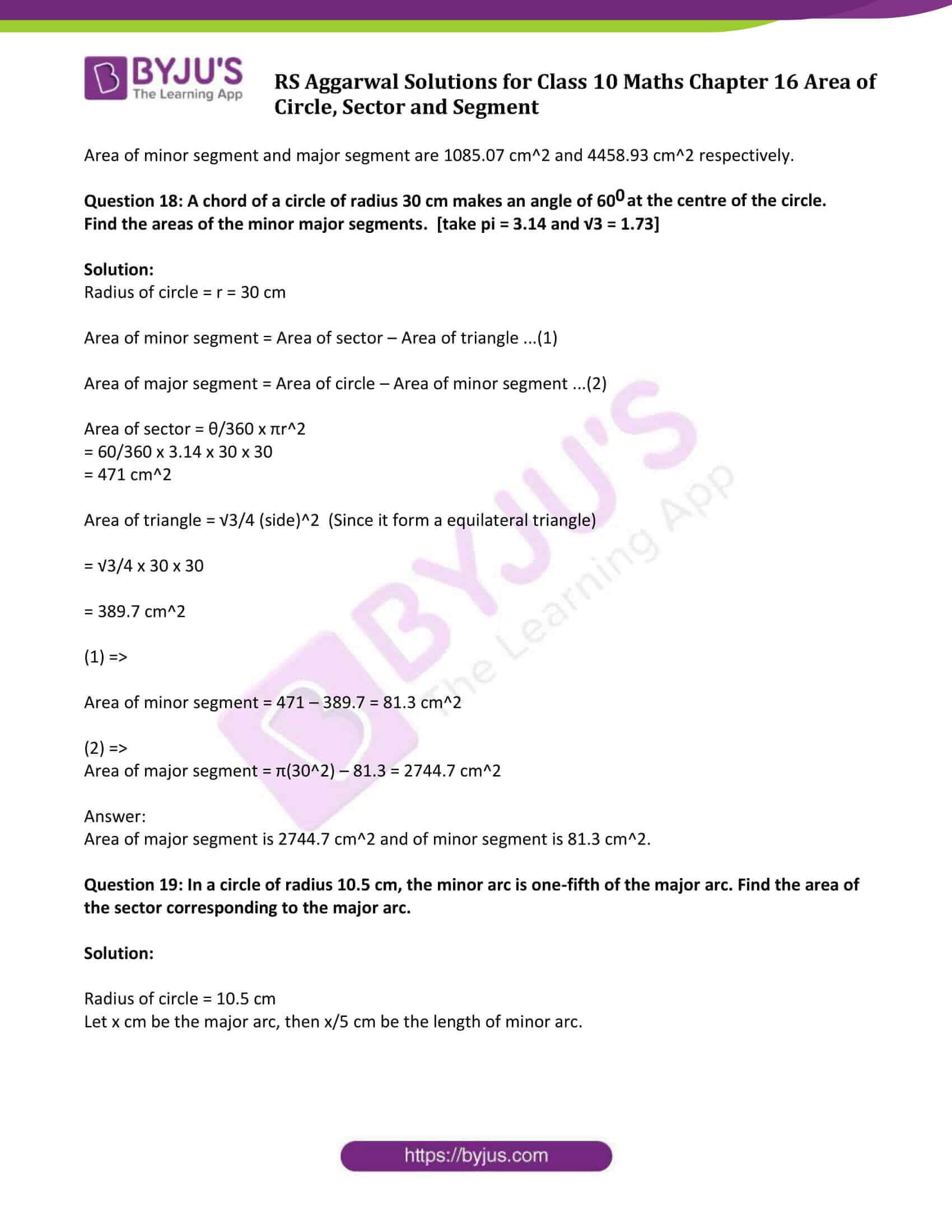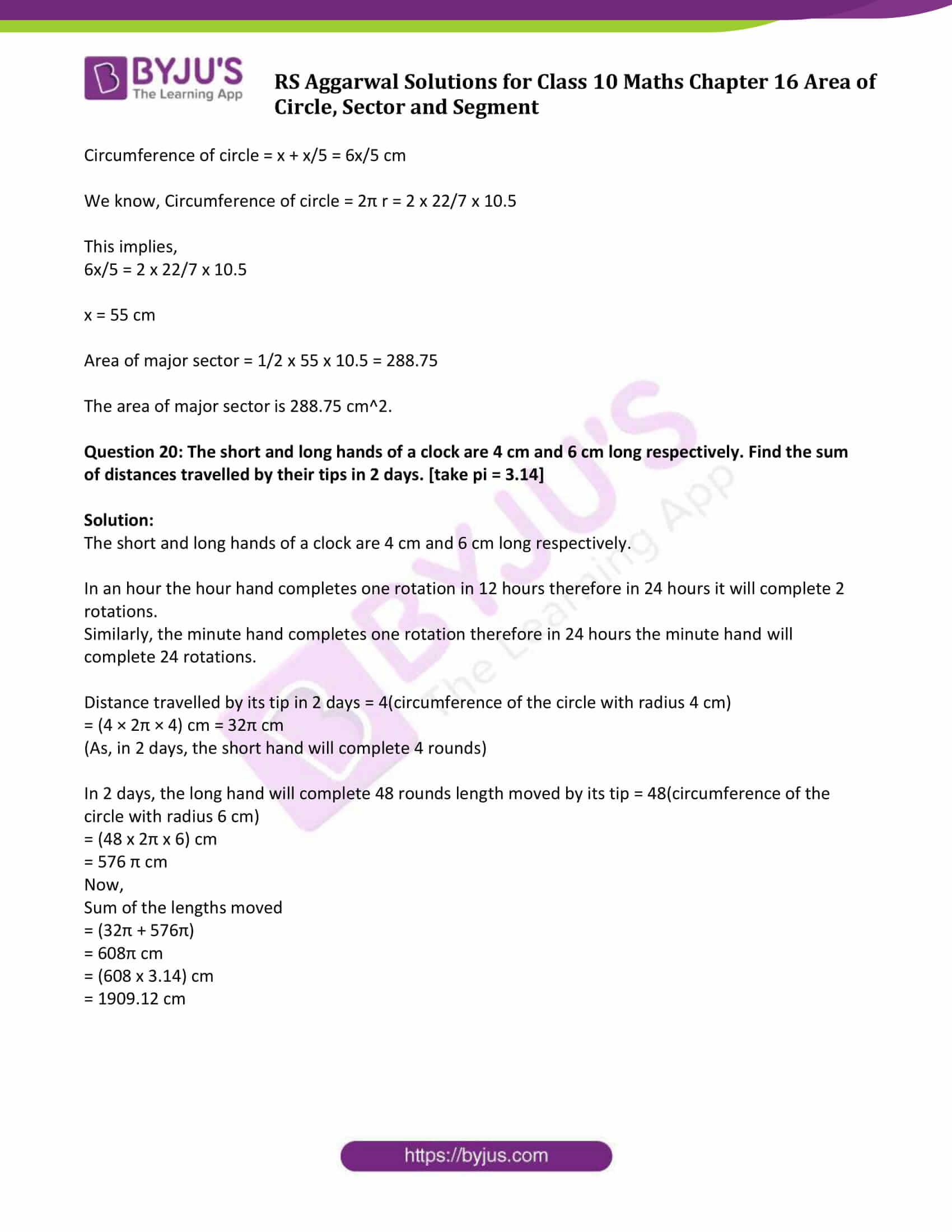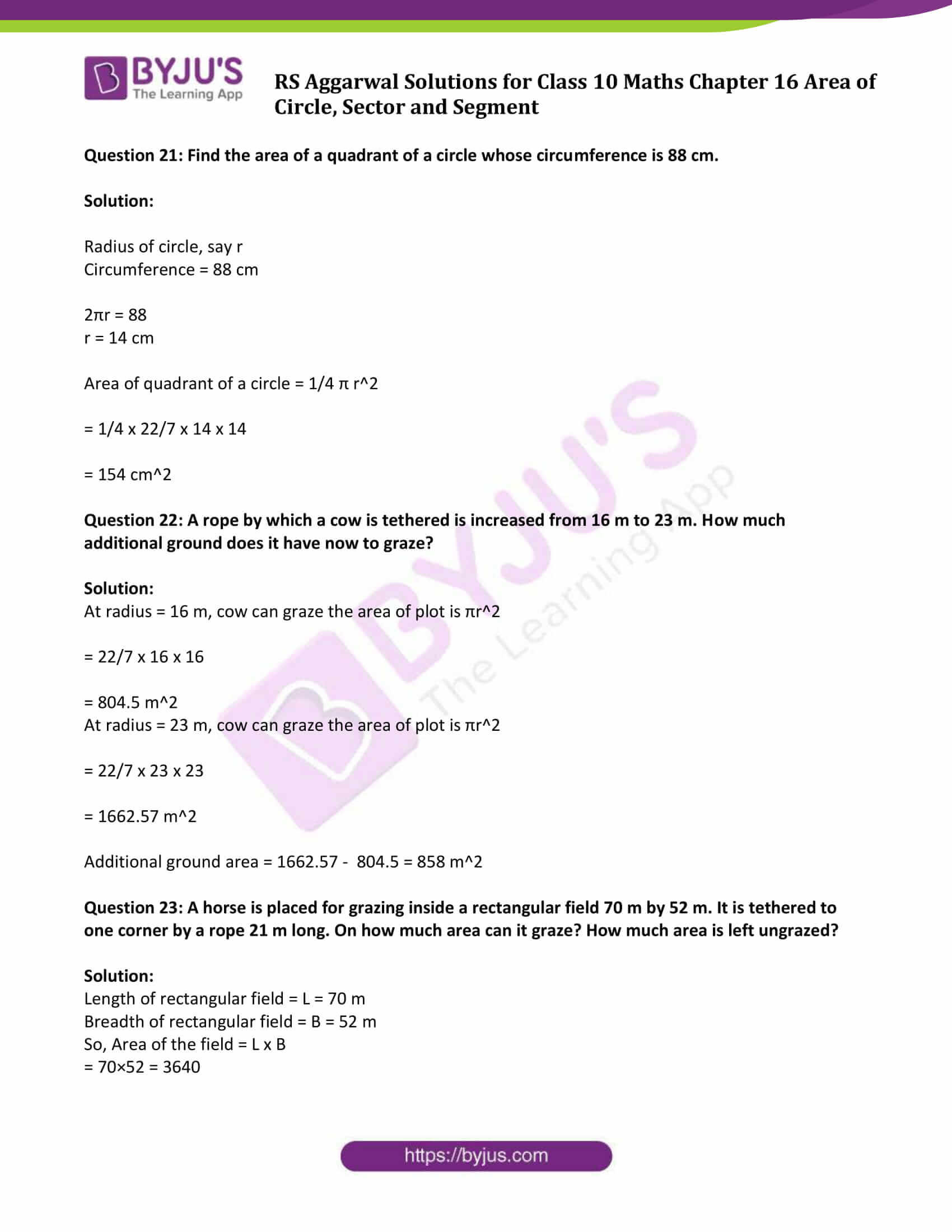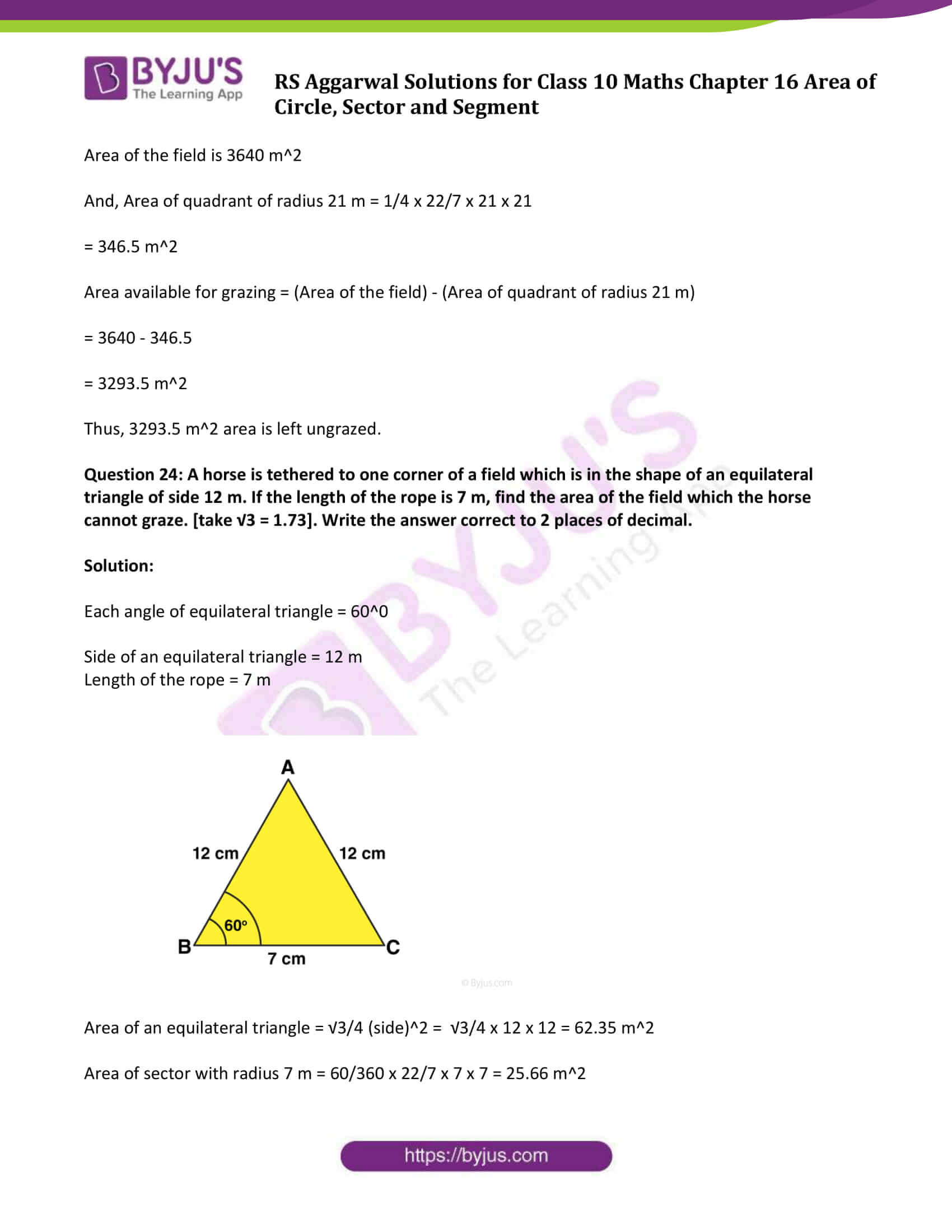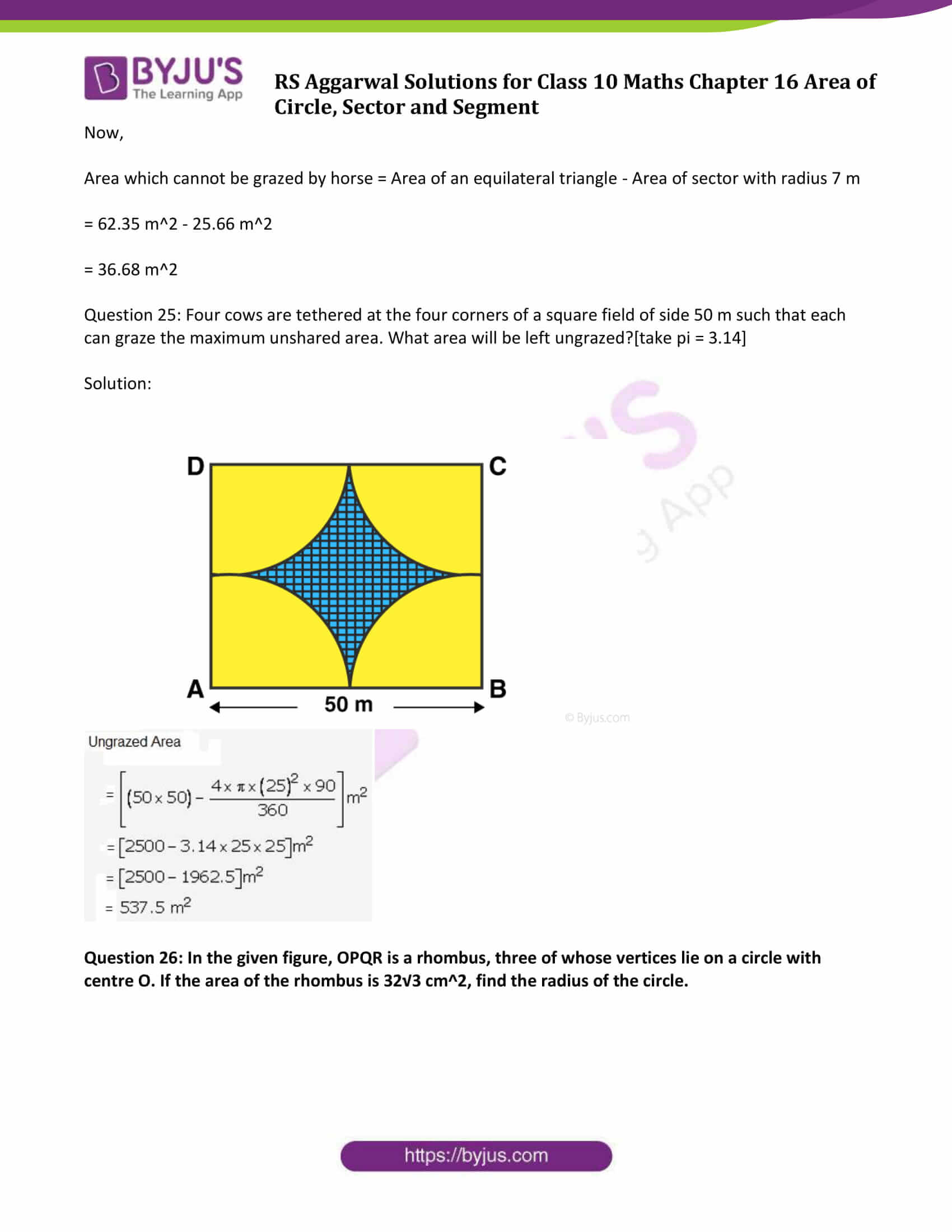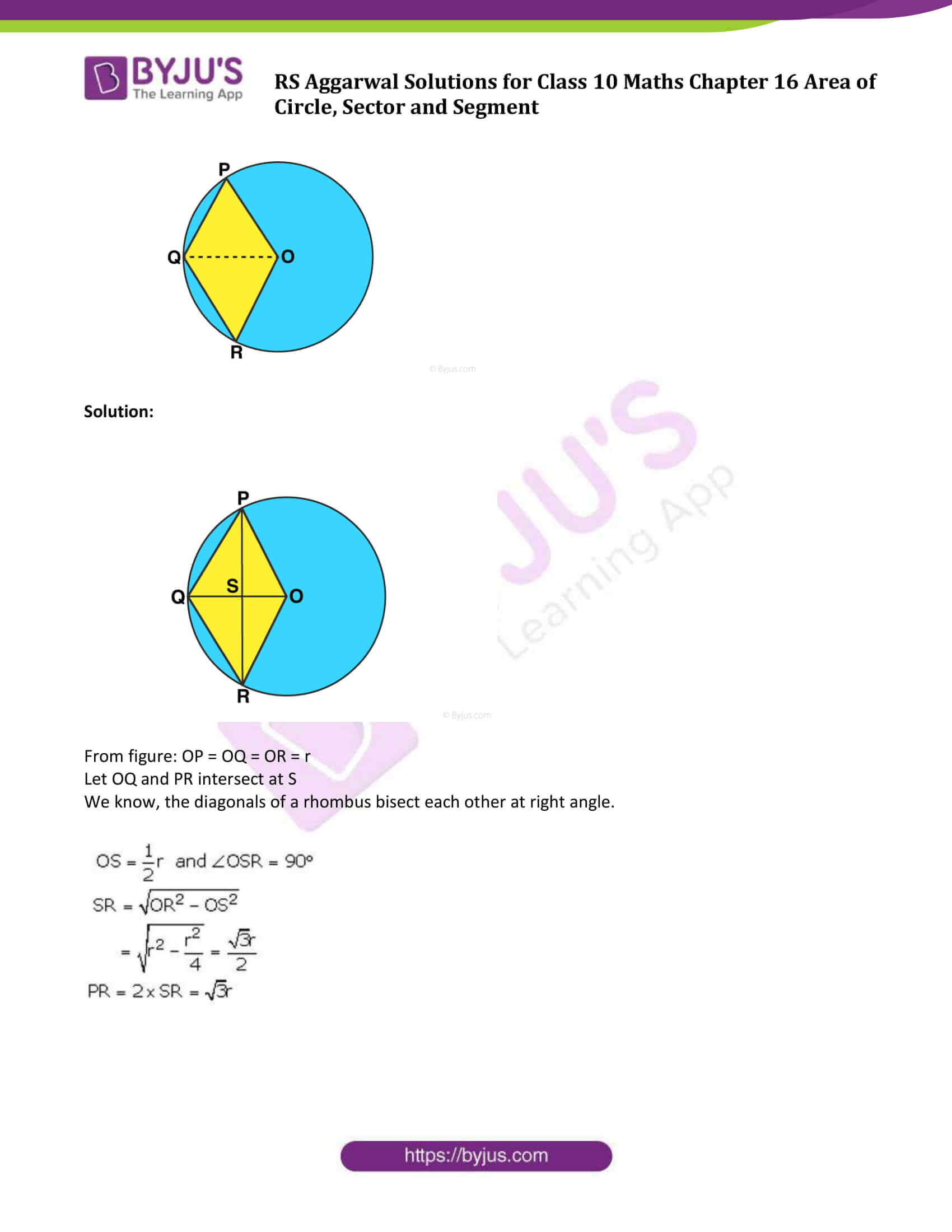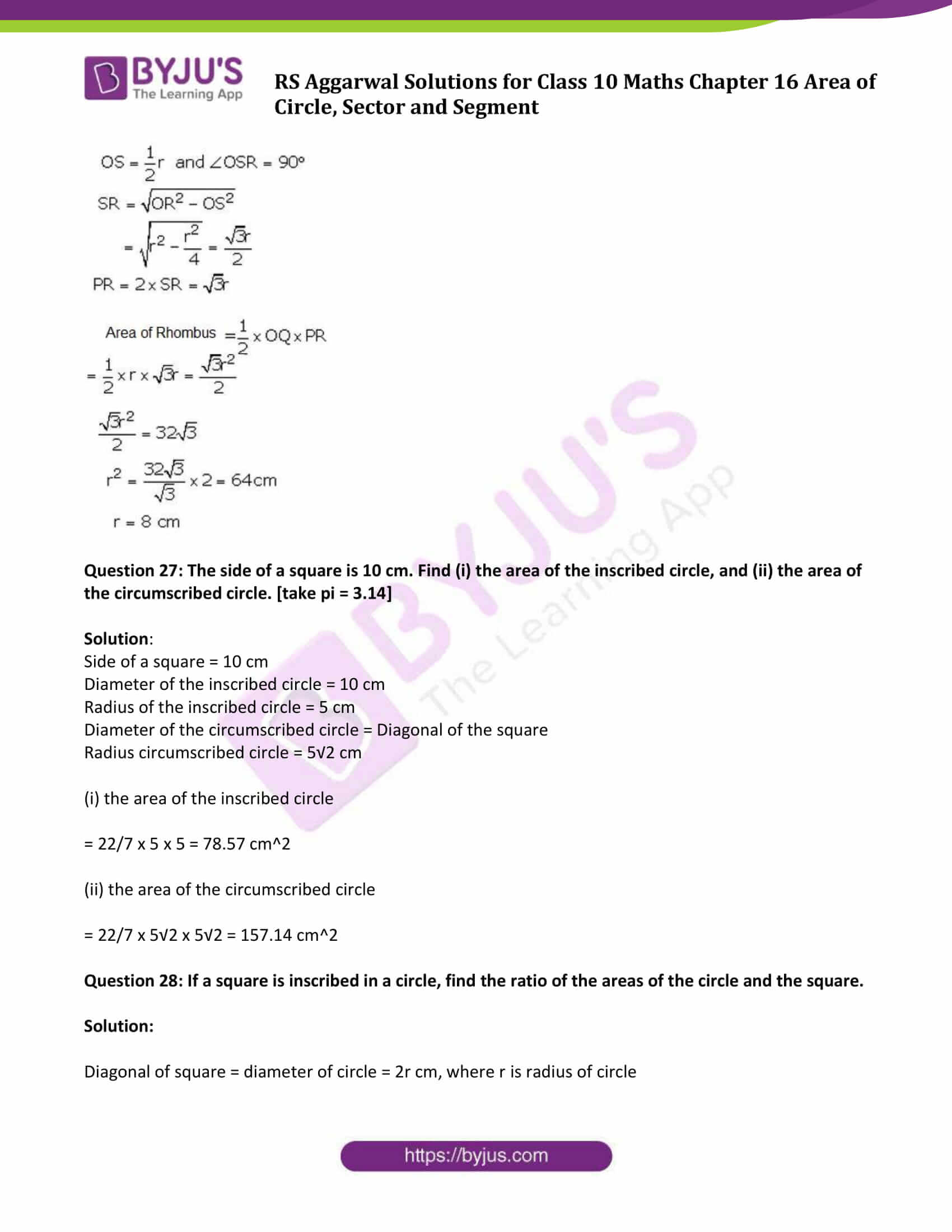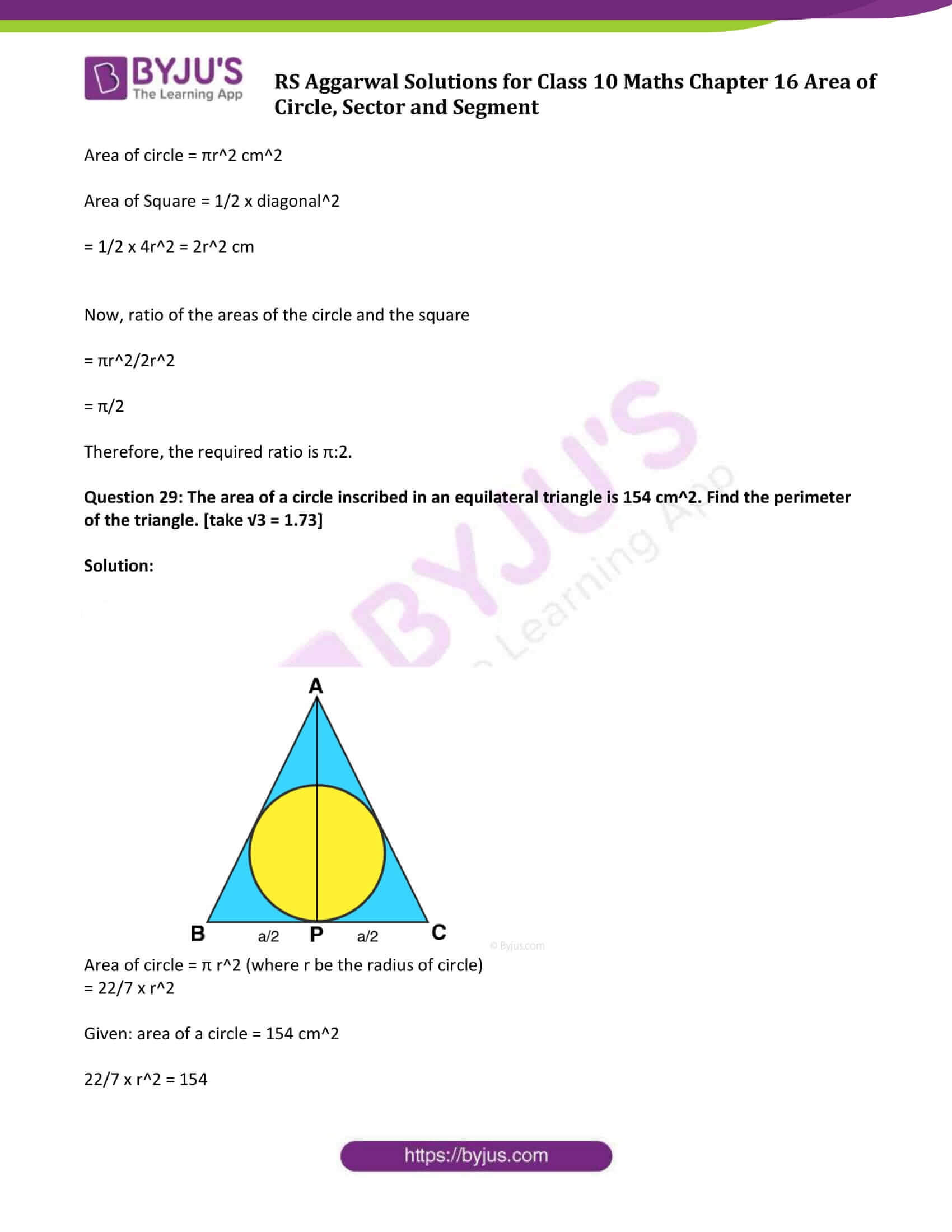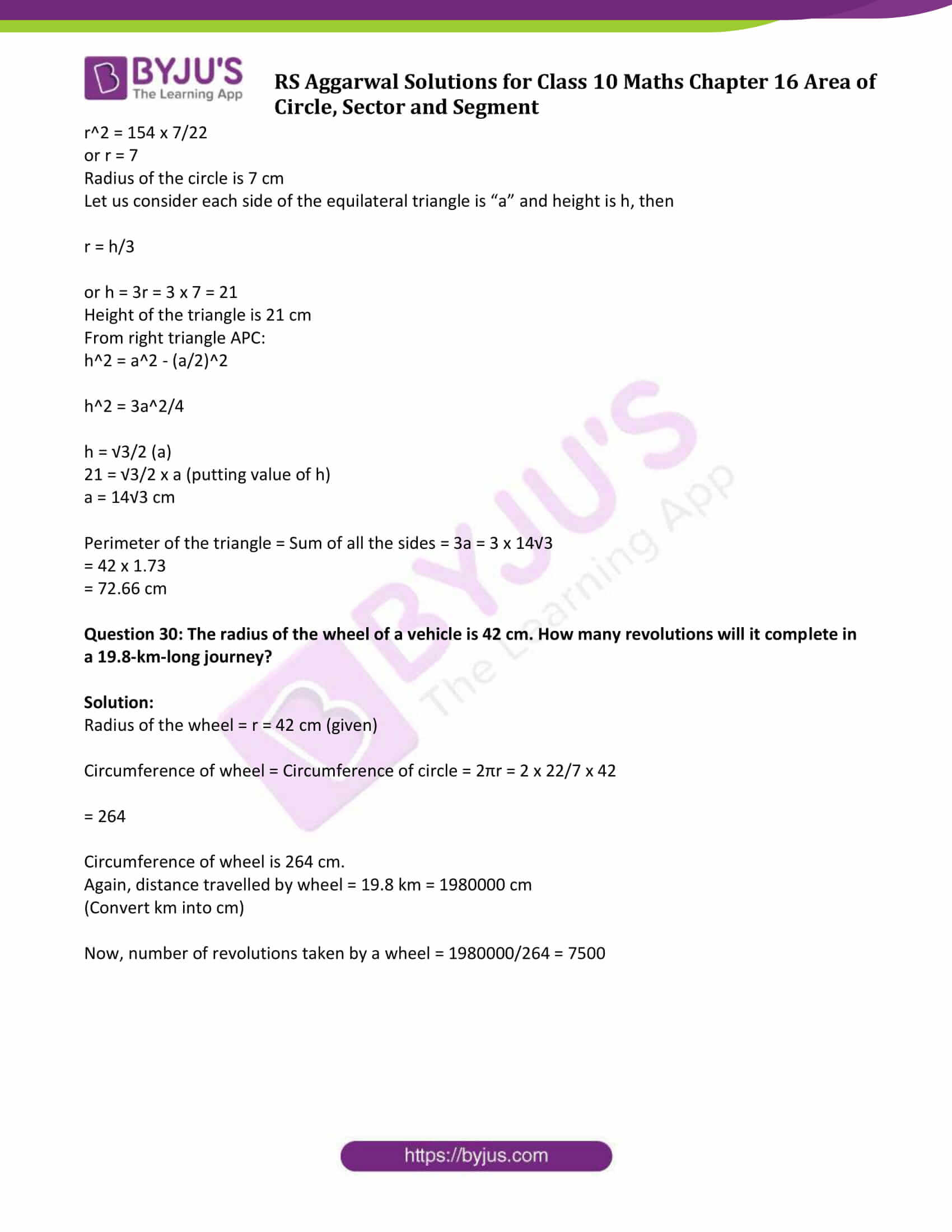### Access other exercise solutions of Class 10 Maths Chapter 16 Area of Circle, Sector and Segment

Exercise 16B Solutions: 27 Questions (Short Answers)

Question 1: The circumference of a circle is 39.6 cm. Find its area.

Solution:

Given: Circumference of the circle = 39.6 cm.

We know, Circumference of circle = 2πr

Where, r = Radius of the circle

⇨ 2πr = 39.6

r = 39.6/2π

r = 6.3

(put value of π = 22/7)

Area of the circle = πr2

Where, r = radius of the circle

⇨ Area of the circle = π(6.3)2

= 124.74

So, area of circle is 124.74 cm2.

Question 2: The area of a circle is 98.56 cm2. Find its circumference.

Solution:

Area of a circle = 98.56 cm2

We know, Area of the circle = πr2

Where, r = radius of the circle

So, πr2 = 98.56

Put π = 22/7

r2 = 98.56/(22/7) = 31.36

r = 5.6 cm

Circumference of circle = 2πr = 2 ×22/7×5.6 = 35.2 cm

Question 3: The circumference of a circle exceeds its diameter by 45 cm. Find the circumference of the circle.

Solution:

Given: circumference of a circle exceeds its diameter by 45 cm.

⇨ Circumference of circle = 45 + Diameter of circle

2π r = 45 + 2r

2r(π – 1) = 45

2r(22/7 – 1) = 45

15/7 r = 45/2

r = 10.5

Circumference of a circle = 2πr = 2 x 22/7 x 10.5 = 66 cm

Question 4: A copper wire when bent in the form of a square encloses an area of 484 cm2. The same wire is not bent in the form of a circle. Find the area enclosed by the circle.

Solution:

Let the square be of side ‘a’ cm and radius of the circle be ‘r’

Area enclosed by the square = 484 cm2

Also, we know that Area of square = Side × Side

Area of the square = a2

⇨ a2 = 484

⇨ a = √484

⇨ a = 22

Therefore, side of square is 22 cm.

Perimeter of square = 4 x side = 4 x 22 = 88

From statement, circumference of the circle = Perimeter of square

2πr = 88

r = (88 x 7)/(2×22) = 14

Radius of the circle = 14 cm

Area of circle = πr2 = 22/7 x 14 x 14 = 616 cm2.

Question 5: A wire when bent in the form of an equilateral triangle encloses an area of 121√3 cm2 . The same wire is bent to form a circle. Find the area enclosed by the circle.

Solution:

Area of an equilateral triangle = 121√3 cm2 (given)

We know that, Area of an equilateral triangle = √3/4 (a2), where “a” is the side of a triangle.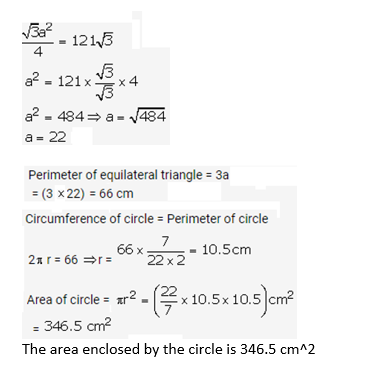The area enclosed by the circle is 346.5 cm2

Question 6: The length of a chain used as the boundary of a semicircular park is 108 m. Find the area of the park.

Solution:

The length of a chain used as the boundary of a semicircular park = 108 m

i.e. circumference of a semicircular park = 108 m

Let the radius of semicircular park = r

Which implies,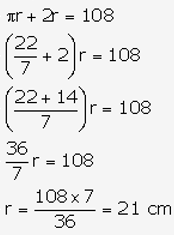Now,

Area of a semicircular park = πr2 = 22/7 x 21 x 21 = 1386

Area of the park = 1/2 (area of semicircular park) = 1/2 x 1386 = 693

Therefore, Area of the park is 693 m2.

Question 7: The sum of the radii of two circles is 7 cm, and the difference of their circumferences is 8 cm. Find the circumference of the circles.

Solution:

The sum of the radii of two circles is 7 cm (given)

Difference of circumferences of two circles is 8 cm (given)

Let radii of circles be x and (7-x)

Then,

2πx – 2π(7 – x) = 8

2πx – 14π + 2πx = 8

2πx = 26 …..(1)

Which is circumference of one circle.

Circumference of the another circles = 2π(7 – x) = 14π – 2πx

= 14π – 26

= 14 x 22/7 – 26

= 18

Therefore, Circumference of the circles are 26 cm and 18 cm.

Question 8: Find the area of a ring whose outer and inner radii are respectively 23 cm and 12 cm.

Solution: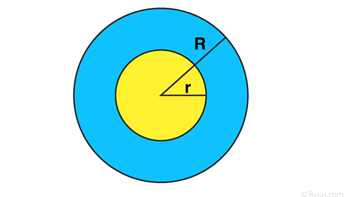Radius of outer ring = R = 23 cm

Radius of inner ring = r = 12 cm

Area of outer circle = πR2

= 22/7 x 23 x 23

= 1662.5 cm2

Area of inner circle = π r2

= 22/7 x 12 x 12

= 452.2 cm2

Area of Ring = outer area – inner area

= 1662.5 – 452.2

= 1210

Area of the ring is 1210 cm2

Question 9:

(i)A path of 8 m width runs around the outsider of a circular park whose radius is 17 m. Find the area of the path.

Solution:

Width of the path = 8 m

Inner radius of the circular park = 17 m

Outer radius of the circular park = (17 + 8) = 25 m

Now,

Area of the path = π[(252 – (172)]

= 22/7 x 42 x 8

= 1056

Area of the path is 1056 m2.

(ii) A park is in the shape of a circle of diameter 7m. It is surrounded by a path of width 0.7m. Find the expenditure of cementing the path at the rate of ₹ 110 per sq.m.

Solution:

Diameter of park = 7 m

so, radius of park = r1 = 3.5 m

Width of path = 0.7 m

Bigger radius of park = r2 = 0.7 + 3.5 = 4.2 m

Now, Area of path = area of bigger circle -area of smaller circle

= Pi r22 – Pi r12

= Pi(r22 – r12)

= 22/7 (4.22– 3.5 2)

= 22/7(17.64 – 12.25)

=16.94 m2

Also,

Cost of expenditure = rate × area

= 110 × 16.94

= 1864.40

Cost of Expenditure of cementing the path is ₹ 1864.40.

Question 10: A racetrack is in the form of a ring whose inner circumference is 352 m and outer circumference is 396 m. Find the width and the area of the track.

Solution:

Let r and R be the radii of inner circle and outer boundaries respectively.

Then,

Circumference of inner circle = 2πr = 352 and

Circumference of outer circle = 2πR = 396

r = 352/2π and R = 396/2π

Width of the track = (R – r) = 396/2π – 352/2π

= 44/2π

= 44/2 x 7/22

= 7

Width of the track is 7 m.

Again, R + r = 396/2π + 352/2π = 748/2π

Area of the track = π(R2 – r2)

= π(R – r) (R+r)

= π x 7 x 748/2π

= 2618

Therefore, the area of the track is 2618 m2.

Question 11: A sector is cut from a circle of radius 21 cm. The angle of the sector is 1500. Find the length of the arc and the area of the sector.

Solution: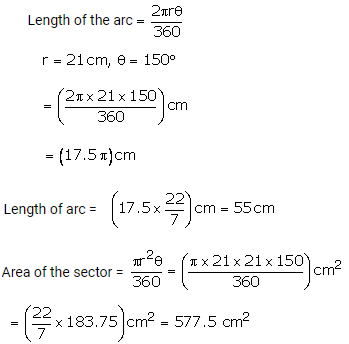Question 12: A chord PQ of the circle of radius 10 cm subtends an angle of 60 degrees at the centre of the circle. Find the area of minor and major segment of the circle.

Solution: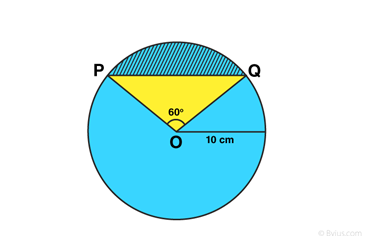The radius of the circle = 10 cm.

Chord PQ subtends an angle = 60 degrees at the centre.

PQO is an equilateral triangle, O being the centre of the circle.

Area of minor segment = θ/360 x π r2 – 1/2 r2 sinθ

= 60/360 x 22/7 x 10 x 10 – 1/2 x 10 x 10 x √3/2

= 9.03

Area of circle = π r2 = 22/7 x 10 x 10 = 314 (approx.)

Area of minor segment = Area of circle – Area of minor segment

= 314 – 9.03

= 304.97 cm2 (approx.)

Question 13: The length of an arc of a circle, subtending an angle of 540 at the centre, is 16.5 cm. Calculate the radius, circumference and area of the circle.

Solution: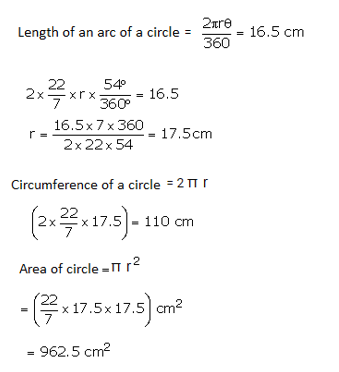Question 14: The radius of a circle with centre O is 7 cm. Two radii OA and OB are drawn at right angles to each other. Find the areas of minor and major segments.

Solution:

Radius of a circle = 7 cm

Area of circle = πr2 = 22/7 x 7 x 7 = 154 cm2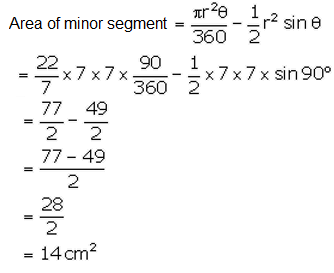Area of major segment = Area of circle – Area of minor segment

= 154 – 14

= 140

Therefore, Area of major segment is 140 cm2.

Question 15: Find the lengths of the arcs cut off from a circle of radius 12 cm by a chord 12 cm long. Also, find the area of the minor segment. [take pi = 3.14 and √3 = 1.73]

Solution:

The lengths of the arcs cut off from a circle of radius (r ) 12 cm by a chord 12 cm long.

Which shows it form a equilateral triangle POQ, and ∠POQ = 60 degrees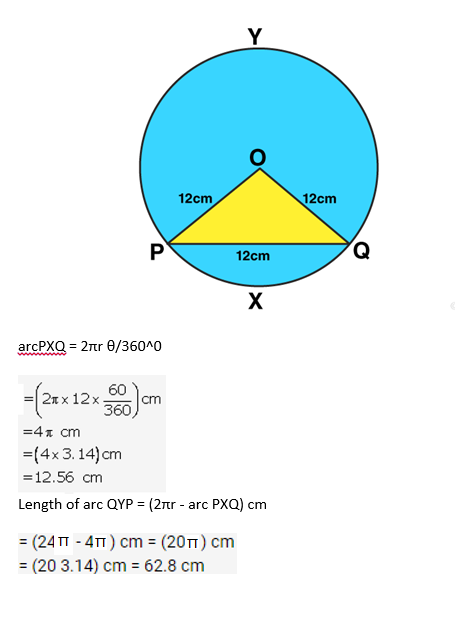Area of minor segment = Area of sector – Area of triangle …(i)

Area of triangle = √3/4 (side)2

= √3/4 (12)2

= 62.28 cm2

Area of sector = θ/360 x πr2

= 60/360 x 3.14 x 12 x 12

= 75.36 cm2

Equation (1) implies

Area of minor segment = 75.36 – 62.28 = 13.08 cm2

Therefore, length of major arc is 62.8 cm and of minor arc is 12.56 cm and area of minor segment is 13.08 cm2.

Question 16: A chord 10 cm long is drawn in a circle whose radius is 5√2 cm. Find the areas of both the segments.[take pi = 3.14]

Solution:

Radius of circle = 5√2 cm

Chord = 10 cm

To find: Areas of both the segments

Now,

Area of minor segment = (area of sector OACBO) – (area of ∆OAB)

= 39.25 – 25 = 14.25 cm2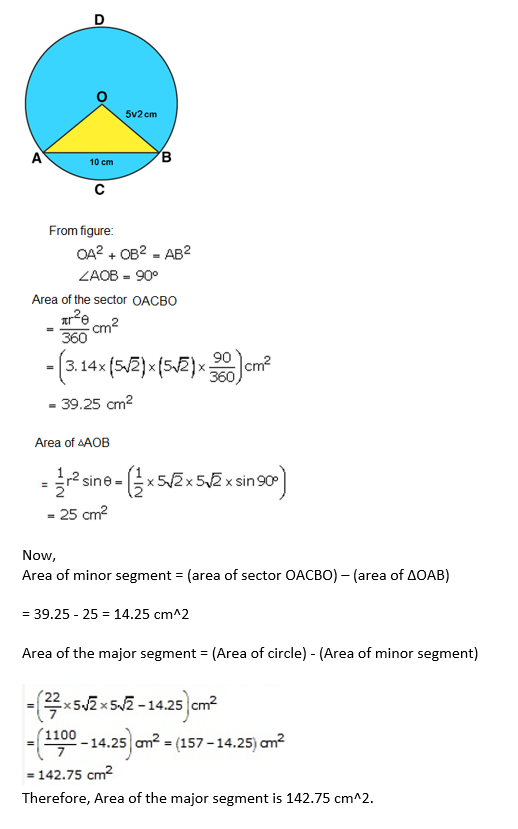Question 17: Find the areas of both the segments of a circle of radius 42 cm with central angle 1200. [ given sin 1200 = √3/2 and √3 = 1.73 ]

Solution: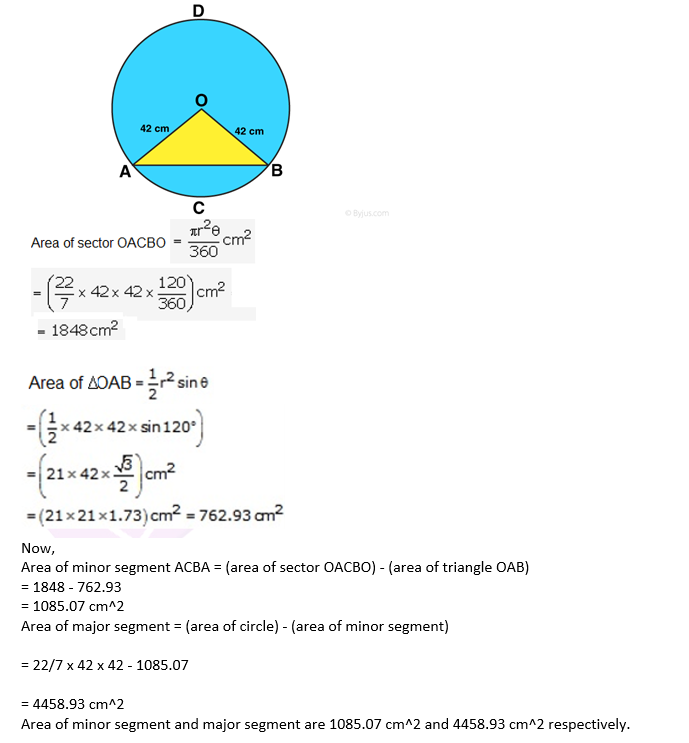Question 18: A chord of a circle of radius 30 cm makes an angle of 600 at the centre of the circle. Find the areas of the minor major segments. [take pi = 3.14 and √3 = 1.73]

Solution:

Radius of circle = r = 30 cm

Area of minor segment = Area of sector – Area of triangle …(1)

Area of major segment = Area of circle – Area of minor segment …(2)

Area of sector = θ/360 x πr2

= 60/360 x 3.14 x 30 x 30

= 471 cm2

Area of triangle = √3/4 (side)2 (Since it form a equilateral triangle)

= √3/4 x 30 x 30

= 389.7 cm2

(1) ⇨

Area of minor segment = 471 – 389.7 = 81.3 cm2

(2) ⇨

Area of major segment = π(302) – 81.3 = 2744.7 cm2

Area of major segment is 2744.7 cm2 and of minor segment is 81.3 cm2.

Question 19: In a circle of radius 10.5 cm, the minor arc is one-fifth of the major arc. Find the area of the sector corresponding to the major arc.

Solution:

Radius of circle = 10.5 cm

Let x cm be the major arc, then x/5 cm be the length of minor arc.

Circumference of circle = x + x/5 = 6x/5 cm

We know, Circumference of circle = 2π r = 2 x 22/7 x 10.5

This implies,

6x/5 = 2 x 22/7 x 10.5

x = 55 cm

Area of major sector = 1/2 x 55 x 10.5 = 288.75

The area of major sector is 288.75 cm2.

Question 20: The short and long hands of a clock are 4 cm and 6 cm long respectively. Find the sum of distances travelled by their tips in 2 days. [take pi = 3.14]

Solution:

The short and long hands of a clock are 4 cm and 6 cm long respectively.

In an hour the hour hand completes one rotation in 12 hours therefore in 24 hours it will complete 2 rotations.

Similarly, the minute hand completes one rotation therefore in 24 hours the minute hand will complete 24 rotations.

Distance travelled by its tip in 2 days = 4(circumference of the circle with radius 4 cm)

= (4 × 2π × 4) cm = 32π cm

(As, in 2 days, the short hand will complete 4 rounds)

In 2 days, the long hand will complete 48 rounds length moved by its tip = 48(circumference of the circle with radius 6 cm)

= (48 x 2π x 6) cm

= 576 π cm

Now,

Sum of the lengths moved

= (32π + 576π)

= 608π cm

= (608 x 3.14) cm

= 1909.12 cm

Question 21: Find the area of a quadrant of a circle whose circumference is 88 cm.

Solution:

Circumference = 88 cm

2πr = 88

r = 14 cm

Area of quadrant of a circle = 1/4 π r2

= 1/4 x 22/7 x 14 x 14

= 154 cm2

Question 22: A rope by which a cow is tethered is increased from 16 m to 23 m. How much additional ground does it have now to graze?

Solution:

At radius = 16 m, cow can graze the area of plot is πr2

= 22/7 x 16 x 16

= 804.5 m2

At radius = 23 m, cow can graze the area of plot is πr2

= 22/7 x 23 x 23

= 1662.57 m2

Additional ground area = 1662.57 – 804.5 = 858 m2

Question 23: A horse is placed for grazing inside a rectangular field 70 m by 52 m. It is tethered to one corner by a rope 21 m long. On how much area can it graze? How much area is left ungrazed?

Solution:

Length of rectangular field = L = 70 m

Breadth of rectangular field = B = 52 m

So, Area of the field = L x B

= 70×52 = 3640

Area of the field is 3640 m2

And, Area of quadrant of radius 21 m = 1/4 x 22/7 x 21 x 21

= 346.5 m2

Area available for grazing = (Area of the field) – (Area of quadrant of radius 21 m)

= 3640 – 346.5

= 3293.5 m2

Thus, 3293.5 m2 area is left ungrazed.

Question 24: A horse is tethered to one corner of a field which is in the shape of an equilateral triangle of side 12 m. If the length of the rope is 7 m, find the area of the field which the horse cannot graze. [take √3 = 1.73]. Write the answer correct to 2 places of decimal.

Solution:

Each angle of equilateral triangle = 60^0

Side of an equilateral triangle = 12 m

Length of the rope = 7 m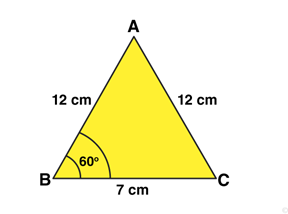Area of an equilateral triangle = √3/4 (side)2 = √3/4 x 12 x 12 = 62.35 m2

Area of sector with radius 7 m = 60/360 x 22/7 x 7 x 7 = 25.66 m2

Now,

Area which cannot be grazed by horse = Area of an equilateral triangle – Area of sector with radius 7 m

= 62.35 m2 – 25.66 m2

= 36.68 m2

Question 25: Four cows are tethered at the four corners of a square field of side 50 m such that each can graze the maximum unshared area. What area will be left ungrazed?[take pi = 3.14]

Solution: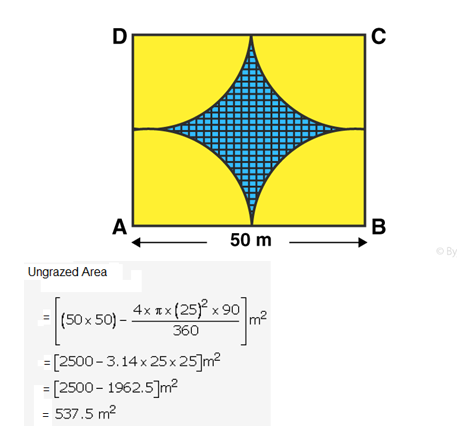Question 26: In the given figure, OPQR is a rhombus, three of whose vertices lie on a circle with centre O. If the area of the rhombus is 32√3 cm2, find the radius of the circle.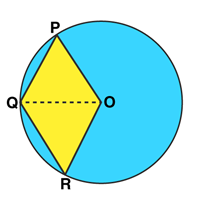Solution: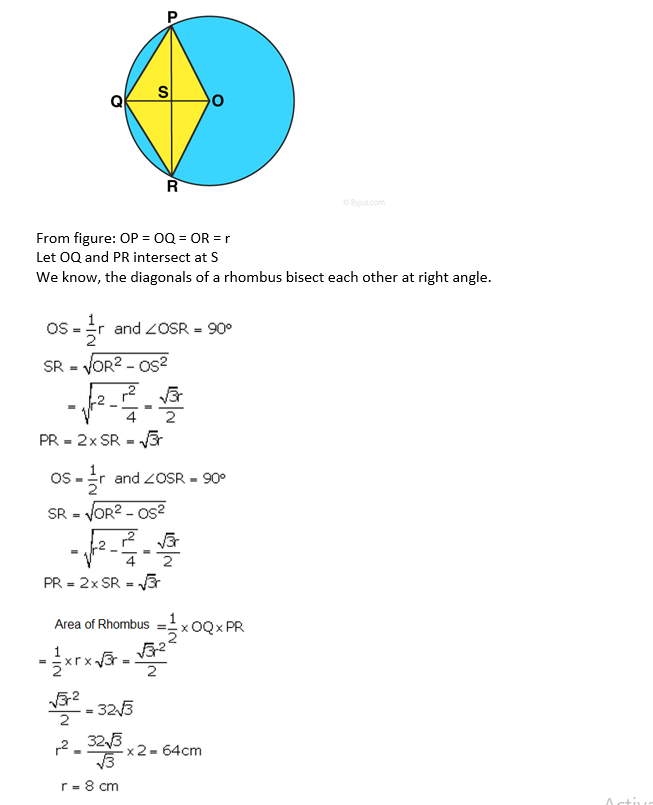Question 27: The side of a square is 10 cm. Find (i) the area of the inscribed circle, and (ii) the area of the circumscribed circle. [take pi = 3.14]

Solution:

Side of a square = 10 cm

Diameter of the inscribed circle = 10 cm

Radius of the inscribed circle = 5 cm

Diameter of the circumscribed circle = Diagonal of the square

Radius circumscribed circle = 5√2 cm

(i) the area of the inscribed circle

= 22/7 x 5 x 5 = 78.57 cm2

(ii) the area of the circumscribed circle

= 22/7 x 5√2 x 5√2 = 157.14 cm2

Question 28: If a square is inscribed in a circle, find the ratio of the areas of the circle and the square.

Solution:

Diagonal of square = diameter of circle = 2r cm, where r is radius of circle

Area of circle = πr2 cm2

Area of Square = 1/2 x diagonal2

= 1/2 x 4r2 = 2r2 cm

Now, ratio of the areas of the circle and the square

= πr2/2r2

= π/2

Therefore, the required ratio is π:2.

Question 29: The area of a circle inscribed in an equilateral triangle is 154 cm2. Find the perimeter of the triangle. [take √3 = 1.73]

Solution: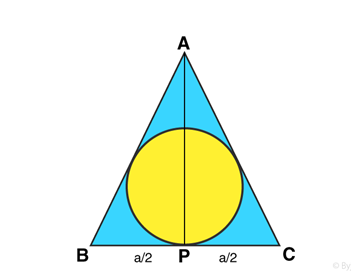Area of circle = π r2 (where r be the radius of circle)

= 22/7 x r2

Given: area of a circle = 154 cm2

22/7 x r2 = 154

r2 = 154 x 7/22

or r = 7

Radius of the circle is 7 cm

Let us consider each side of the equilateral triangle is “a” and height is h, then

r = h/3

or h = 3r = 3 x 7 = 21

Height of the triangle is 21 cm

From right triangle APC:

h2 = a2 – (a/2)2

h2 = 3a2/4

h = √3/2 (a)

21 = √3/2 x a (putting value of h)

a = 14√3 cm

Perimeter of the triangle = Sum of all the sides = 3a = 3 x 14√3

= 42 x 1.73

= 72.66 cm

Question 30: The radius of the wheel of a vehicle is 42 cm. How many revolutions will it complete in a 19.8-km-long journey?

Solution:

Radius of the wheel = r = 42 cm (given)

Circumference of wheel = Circumference of circle = 2πr = 2 x 22/7 x 42

= 264

Circumference of wheel is 264 cm.

Again, distance travelled by wheel = 19.8 km = 1980000 cm

(Convert km into cm)

Now, number of revolutions taken by a wheel = 1980000/264 = 7500

## R S Aggarwal Solutions for Class 10 Maths Chapter 16 Area of Circle, Sector and Segment Exercise 16A Topics

Class 10 Maths Chapter 16 Area of Circle, Sector and Segment Exercise 16A is based on the topics:

• Circle and its parts: circumference, radius, diameter, chord, secant, arc, central angle.
• Semicircle
• Segment
• Sector of a circle